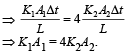Courses

# Test: Thermal Properties Of Matter - From Past 28 Years Questions

## 39 Questions MCQ Test Physics 28 Years Past year papers for NEET/AIPMT Class 11 | Test: Thermal Properties Of Matter - From Past 28 Years Questions

Description
This mock test of Test: Thermal Properties Of Matter - From Past 28 Years Questions for NEET helps you for every NEET entrance exam. This contains 39 Multiple Choice Questions for NEET Test: Thermal Properties Of Matter - From Past 28 Years Questions (mcq) to study with solutions a complete question bank. The solved questions answers in this Test: Thermal Properties Of Matter - From Past 28 Years Questions quiz give you a good mix of easy questions and tough questions. NEET students definitely take this Test: Thermal Properties Of Matter - From Past 28 Years Questions exercise for a better result in the exam. You can find other Test: Thermal Properties Of Matter - From Past 28 Years Questions extra questions, long questions & short questions for NEET on EduRev as well by searching above.
QUESTION: 1

### N molecules each of mass m of a gas  A and 2N molecules each of mass 2m of gas B are contained in the same vessel which is maintained at temperature T. The mean square velocity of molecules of B type is v2 and the mean square rectangular component of the velocity of A type is denoted by ω2. Then ω2/v2 

Solution:

Mean kinetic energy of the two types of molecules should be equal. The mean square velocity of A type molecules = ω2 + ω2 + ω2 = 3ω2

Therefore,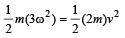This gives ω2 / v2 = 2/3

QUESTION: 2

### 10 gm of  ice  cubes at 0°C are released in a tumbler (water equivalent 55 g) at 40°C.Assuming that negligible heat is taken from the surroundings, the temperature of water in the tumbler becomes nearly (L = 80 cal/g) 

Solution:

Let the final temperature  be T Heat gained by ice  = mL + m × s × (T – 0) = 10 × 80 + 10 × 1 × T
Heat lost by water = 55 × 1× (40 – T)
By using law of calorimetery, 800 + 10 T = 55 × (40 – T)
⇒ T = 21.54°C = 22°C

QUESTION: 3

### A centigrade and a Fahrenheit thermometer are dipped in boiling water. The water temperature is lowered until the Fahrenheit thermometer registers 140°. What is the fall in temperature as registered by the Centigrade thermometer ?

Solution:

Using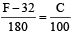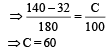Temperature of boiling water = 100°C
We get, fall in temperature = 100 – 60 = 40°C

QUESTION: 4

Thermal capacity of 40 g of aluminium (s = 0.2 cal /g K) is 

Solution:

Thermal capacity = ms = 40 × 0.2 = 8 cal/°C = 4.2 × 8 J = 33.6 joules/°C

QUESTION: 5

Mercury thermometer can be used to measure temperature upto 

Solution:

Mercury thermometer is based on the principle of change of volume with rise of temperature and can measure temperatures ranging from –30°C to 357°C.

QUESTION: 6

If the temperature of the sun is doubled, the rate of energy  received on earth will be increased by a factor of 

Solution:

Amount of energy radiated ∝ T4

QUESTION: 7

A body cools from 50.0°C to 48°C in 5s. How long will it take to cool from 40.0°C to 39°C?Assume the temperature of surroundings to be 30.0°C and Newton's law of cooling to be valid.

Solution: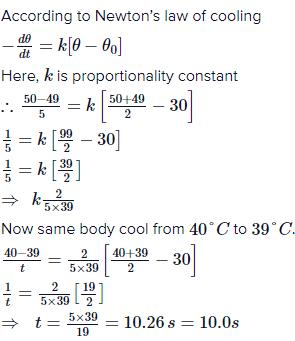QUESTION: 8

A beaker full of hot water is kept in a room. If it cools from 80°C to 75°C in t1 minutes, from 75° C to 70°C in t2 minutes and from 70°C to 65°C in t3 minutes, then 

Solution:

Let θ0 be the temperature of the surrounding.
Then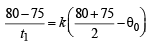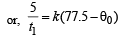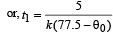… (1)

Similarly,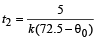…(2)

and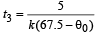… (3)
From (1), (2) & (3), it is obvious that

t1 < t2 < t3

QUESTION: 9

A black body is at temperature of 500 K. It emits energy at rate which is proportional to 

Solution:

According to Stefan's Law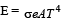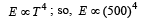QUESTION: 10

The radiant energy from the sun, incident normally at the surface of earth is 20 k cal/m2 min. What would have been the radiant energy, incident normally on the earth, if the sun had a temperature, twice of the present one? 

Solution:

According to Stefan’s law

E ∝ T4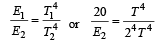⇒ E2 = 320 kcal/m2. min.

QUESTION: 11

If 1 g of steam is mixed with 1 g of ice, then the resultant temperature of the mixture is 

Solution:

Heat required by ice a t 0° C to reach a temperature of 100°C = mL + mcΔθ = 1 × 80 + 1 × 1 × (100 – 0) = 180 cal
Heat available with 1 g steam to condense into 1 g of water at 100°C = 536 cal.
Obviously the whole steam will not be condensed and ice will attain a temperature of 100°C; so the temperature of mixture = 100°C.

QUESTION: 12

The coefficient of linear expansions of brass and steel are α1 and α2 respectively. When we take a brass rod of length ℓ1 and a steel rod of length ℓ2 at 0ºC, then the difference in their lengths (ℓ2 – ℓ1) will remain the same at all temperatures if [1995, 1999]

Solution:

Let ΔT be increase in the temperature of brass wire.Then length of brass wire,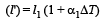Similarly, length of steel wire when temperature is increased by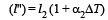According to question , l' – l'' = l1 – l2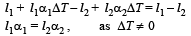QUESTION: 13

The presence of gravitational field is required for the heat transfer by 

Solution:

In convection, the temperature gradient exists in the vertical direction and not in the horizontal direction. So, up and down movement of particles takes place which depends on the weight and gravity.

QUESTION: 14

A black body has maximum wavelength λm at temperature 2000 K. Its corresponding wavelength at temperature 3000 K will be

Solution:

According to Wein's displacement law,

λmT = 2.88 × 10–3 When T = 2000 K,
λm (2000) = 2.88 × 10–3 ....(1)
When T = 3000 K, λ'm (3000) = 2.88 × 10–3 ....(2)
Dividing (1) by (2),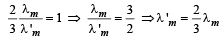QUESTION: 15

A cylindrical rod having temperature T1 and T2 at its end. The rate of flow of heat is Q1 cal/sec.If all the linear dimensions are doubled keeping temperature constant, then the rate of flow of heat Q2 will be 

Solution: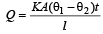Rate of heat flow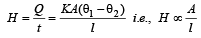Dimensions of area A = [L2], dimensions of distance l = [L]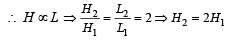QUESTION: 16

Wien's law is concerned with 

Solution:

According to Wein's displacement law, product of wavelength belonging to maximum intensity and temperature is constant i.e.,  λmT = constant.

QUESTION: 17

Radiation from which of the following sources, approximates black body radiation best? 

Solution:

This hole in the cavity is also called Fery's black body, it is made such that when light enters the cavity it suffers multiple reflection inside it and since with every reflection some part is absorbed so almost all the radiant light get absorbed.

QUESTION: 18

Two rods of thermal conductivities K1 and K2, cross-sections A1 and A2 and specific heats S1 and S2 are of equal lengths. The temperatures of two ends of each rod are T1 and T2. The rate of flow of heat at the steady state will be equal if 

Solution:

Rate of heat flow for one rod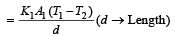.Rate of heat flow for other rod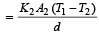In steady state,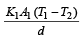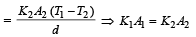QUESTION: 19

Consider a compound slab consisting of two different materials having equal thicknesses and thermal conductivities K and 2K, respectively.
The equivalent thermal conductivity of the slab is                  

Solution:

In series, equivalent thermal conductivity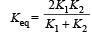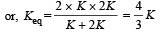QUESTION: 20

If λm denotes the wavelength at which the radiative emission from a black body at a temperature T K is maximum, then 

Solution:

From Wein’s displacement law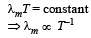QUESTION: 21

Which of the following circular rods (given radius r and length l), each made of the same material and whose ends are maintained at the same temperature will conduct most heat? 

Solution:

We know that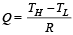Also, Thermal resistance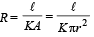Heat flow will be maximum when thermal resistance is minimum.
From given option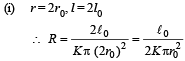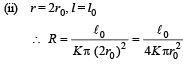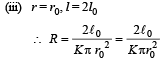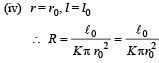It is clear that for option (2) resistance is minimum, hence, heat flow will be maximum. [Alt : (i) Rate of heat flow is directly proportional to area
(ii) inversely proportional to length.

∴ Heat flow will be maximum when r is maximum and ℓ is minimum. ]

QUESTION: 22

The temperature of in version of a thermocouple is 6200C and the neutral temperature is 3000C. What is the temperature of cold junction ? 

Solution: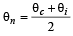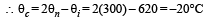QUESTION: 23

A black body at 1227°C emits radiations with maximum intensity at a wavelength of 5000Å. If the temperature of the body is increased by 1000°C, the maximum intensity will be observed at

Solution:

Applying Wein's displacement law, λmT = constant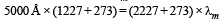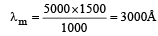QUESTION: 24

A black body is at 727° C. It emits energy at a rate which is proportional to 

Solution:

According to Stefan's law, E ∝ T4

∝ (t + 273)4 K              [727°C = (727 + 273)K]
∝ (727 + 273)4 K
∝ (1000)4 K

QUESTION: 25

If the cold junction of a thermo-couple is kept at 0°C and the hot junction is kept at T°C then the relation between neutral temperature (Tn) and temperature of inversion (Ti) is 

Solution:

Since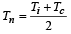= Neutral temperature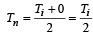[Tc = 0°C = temperature of cold junction]

QUESTION: 26

Assuming the sun to have a sph erical outer surface of radius r, radiating like a black body at temperature t°C, the power received by a unit surface, (normal to the incident rays) at a distance R from the centre of the sun is        where σ is the Stefan’s constant.

Solution:

Power radiated by the sun at t°C

= σ(t + 273)44πr2

Power received by a unit surface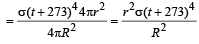QUESTION: 27

On a new scale of temperature (which is linear) and called the W scale, the freezing and boiling points of water are 39° W and 239° W respectively. What will be the temperature on the new scale, corresponding to a temperature of 39° C on the Celsius scale? 

Solution:

For different temperature scales, we have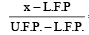= constant

Where L.F.P ⇒  Lower Fixed point
U.H.F. ⇒  Upper fixed point
where x is the measurement at that scale.
Here, if C and W be the measurements on Celsius and W scale then,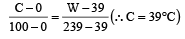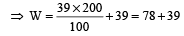= 117° W

QUESTION: 28

An electric kettle takes 4A current at 220 V. How much time will it take to boil 1 kg of water from temperature 20° C? The temperature of boiling water is 100° C. 

Solution:

Heat required to raise the temperature of 1kg water from 20°C to 100°C is given by Q = msΔθ = (1× 4200 × 80) J
Power of kettle (P) = VI = (220 × 4)W

∴ Time taken =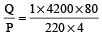= 381.81 sec = 6.36 min

QUESTION: 29

The two ends of a rod of  length L and a uniform cross-sectional area A are kept at two temperatures T1 and T2 (T1 > T2). The rate of heat transfer,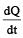through the rod in a steady state is given by: 

Solution: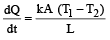[(T1–T2) is the temperature difference]

QUESTION: 30

A black body at 227°C radiates heat at the r ate of 7 cals/cm2s. At a temperature  of 727°C, the rate of heat radiated in the same units will be:

Solution:

Accordin g to Stefan’s law E = σT4,
T1 = 500 K
T2 = 1000 K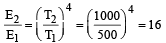∴     E2 = 16 × 7 = 112 cal / cm2s

QUESTION: 31

A cylindrical metallic rod in therrnal contact with two reservoirs of heat at its two ends conducts an amount of heat Q in time t. The metallic rod is melted and the material is formed into a rod of half the radius of the original rod. What is the amount of heat conducted by the new rod, when placed in thermal contact with the two reservoirs in time t? 

Solution:

The rate of heat flow is given by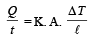Area of Original rod A = πR2 ;

Areal of new rod A' =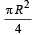Volume of original rod will be equal to the volume of new rod.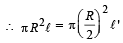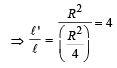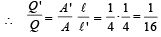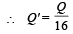QUESTION: 32

The total radiant energy per unit area, normal to the direction of incidence, received at a distance R from the centre of a star of radius r, whose outer surface radiates as a black body at a temperature T K is given by: 

Solution: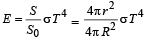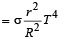QUESTION: 33

If the radius of a star is R and it acts as a black body, what would be the temperature of the star, in which the rate of energy production is Q ?  (σ stands for Stefan’S constant)

Solution:

Stefan’s law for black body radiation Q = σe AT4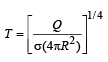Here e = 1
A = 4πR2

QUESTION: 34

Liquid oxygen at 50 K is heated to 300 K at constant pressure of 1 atm. The rate of heating is constant. Which one of the following graphs represents the variation of temperature with time? 

Solution:

Initially liquid oxygen will gain the temperature up to its boiling temperature then it change its state to gas. After this again its temperature will increase, so corresponding graph will be

QUESTION: 35

A slab of stone of area 0.36 m2 and thickness 0.1 m is exposed on the lower surface to steam at 100°C. A block of ice at 0°C rests on the upper surface of the slab. In one hour 4.8 kg of ice is melted. The thermal conductivity of slab is : (Given latent heat of fusion of ice = 3.36 × 105 Jkg–1.) : [2012M]

Solution: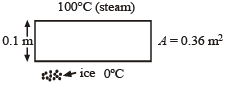Rate of heat given by steam = Rate of heat taken by ice where
K = Thermal conductivity of the slab
m = Mass of the ice
L = Latent heat of melting/fusion
A = Area of the slab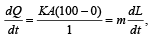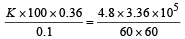K =1.24 J/m/s/°C

QUESTION: 36

A piece of iron is heated in a flame. It first becomes dull red then becomes reddish yellow and finally turns to white hot. The correct explanation for the above observation is possible by using [NEET 2013]

Solution:

Wein’s displacement law According to this law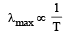or,  λmax × T = constant
So, as the temperature increases λ decreases.

QUESTION: 37

The molar specific heats of an ideal gas at constant pressure and volume are denoted by Cp and Cv, respectively. If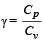and R is theuniversal gas constant, then Cv is equal to [NEET 2013]

Solution: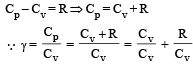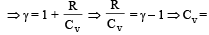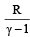QUESTION: 38

The density of water at 20°C is 998 kg/m3 and at 40°C 992 kg/m3. The coefficient of volume expansion of water is [NEET Kar. 2013]

Solution:

From question, Δρ = (998 – 992) kg/m3 = 6 kg/m3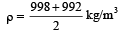= 995 kg/m3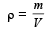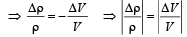∴ Coefficient of volume expansion of water,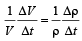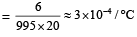QUESTION: 39

Two metal rods 1 and 2 of same lengths have same temperature difference between their ends.Their thermal conductivities are K1 and K2 and cross sectional areas A1 and A2, respectively. If the rate of heat conduction in rod 1 is four times that in rod 2, then [NEET Kar. 2013]

Solution:

Q1 = 4Q2 (Given)